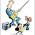## Sunday, November 07, 2010

### SAT questions

Great! New resource for problems. I bought an SAT math only workbook, and it's chock chock CHOCK full of geometry problems asked in all sorts of cool ways. There are a ton of triangle problems using "angles sum to 180" facts and parallel lines and transversal problems.

Here's an example: picture triangle ABC. Then picture point D on segment BC. So you have your larger triangle separated into 2 smaller triangles. Put variables representing 5 of the 6 triangle angles (a,b,c,x,y). Let the 6th angle be 50 degrees. The question asks find a+b+c+x+y.

Love it.

1.OK, so what is the name of the book?

2.Mine is at school, but I'm guessing any one of these is good (I vaguely remember an orange cover, but don't remember the cube)...

http://www.amazon.com/s/ref=nb_sb_ss_i_5_16?url=search-alias%3Dstripbooks&field-keywords=sat+math+level+1&sprefix=sat+math+level+1

3.I am truly weak at these segmented question problems. More the practice, more reliable is the results in exams. All the major comprehensive LSAT Prep Courses are helpful for students in attaining their goals. Main is the effective methodology with superior techniques of solving the questions.

4.Anonymous9:26 AM

5.